# DAV Class 3 Maths Chapter 11 Worksheet 5 Solutions

The DAV Maths Book Class 3 Solutions and DAV Class 3 Maths Chapter 11 Worksheet 5 Solutions of Fractions offer comprehensive answers to textbook questions.

## DAV Class 3 Maths Ch 11 WS 5 Solutions

Question 1.
Find the difference.

(a) $$\frac{7}{9}-\frac{2}{9}$$
Solution:
$$\frac{7}{9}-\frac{2}{9}$$
= $$\frac{7-2}{9}$$
= $$\frac{5}{9}$$

(b) $$\frac{7}{10}-\frac{4}{10}$$
Solution:
$$\frac{7}{10}-\frac{4}{10}$$
= $$\frac{7-4}{10}$$
= $$\frac{3}{10}$$

(c) $$\frac{5}{8}-\frac{3}{8}$$
Solution:
$$\frac{5}{8}-\frac{3}{8}$$
= $$\frac{5-3}{8}$$
= $$\frac{2}{8}$$(d) $$\frac{5}{7}-\frac{1}{7}$$
Solution:
$$\frac{5}{7}-\frac{1}{7}$$
= $$\frac{5-1}{7}$$
= $$\frac{4}{7}$$

(e) $$\frac{4}{5}-\frac{3}{5}$$
Solution:
$$\frac{4}{5}-\frac{3}{5}$$
= $$\frac{4-3}{5}$$
= $$\frac{1}{5}$$

(f) $$\frac{8}{9}-\frac{3}{9}$$
Solution:
$$\frac{8}{9}-\frac{3}{9}$$
= $$\frac{8-3}{9}$$
= $$\frac{5}{9}$$Question 2.
Subtract the following fractions:

(a) $$\frac{2}{8}$$ from $$\frac{6}{8}$$
Solution:
$$\frac{6}{8}-\frac{2}{8}$$
= $$\frac{6-2}{8}$$
= $$\frac{4}{8}$$

(b) $$\frac{8}{9}$$ from $$\frac{9}{9}$$
Solution:
$$\frac{9}{9}-\frac{8}{9}$$
= $$\frac{9-8}{9}$$
= $$\frac{1}{9}$$

(c) $$\frac{3}{7}$$ from $$\frac{6}{7}$$
Solution:
$$\frac{6}{7}-\frac{3}{7}$$
= $$\frac{6-3}{7}$$
= $$\frac{3}{7}$$(d) $$\frac{3}{8}$$ from $$\frac{7}{8}$$
Solution:
$$\frac{7}{8}-\frac{3}{8}$$
= $$\frac{7-3}{8}$$
= $$\frac{4}{8}$$

(e) $$\frac{1}{10}$$ from $$\frac{9}{10}$$
Solution:
$$\frac{9}{10}-\frac{1}{10}$$
= $$\frac{9-1}{10}$$
= $$\frac{8}{10}$$

(f) $$\frac{2}{5}$$ from $$\frac{4}{5}$$
Solution:
$$\frac{4}{5}-\frac{2}{5}$$
= $$\frac{4-2}{5}$$
= $$\frac{2}{5}$$Question 3.
Colour squares to show the correct answer.

(a) $$\frac{3}{5}-\frac{1}{5}$$ =Solution:

$$\frac{2}{5}$$ =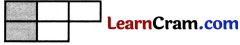(b) $$\frac{1}{4}-\frac{1}{4}$$ =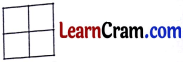Solution:

0 =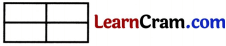(c) $$\frac{7}{9}-\frac{2}{9}$$ =Solution:

$$\frac{5}{9}$$ =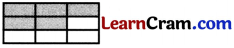(d) $$\frac{3}{3}-\frac{2}{3}$$ =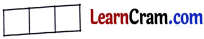Solution:

$$\frac{1}{3}$$ =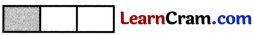(e) $$\frac{5}{10}-\frac{4}{10}$$ =Solution:

$$\frac{1}{10}$$ =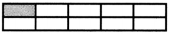(f) $$\frac{9}{9}-\frac{6}{9}$$ =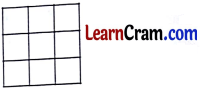Solution:

$$\frac{3}{9}$$ =### DAV Class 3 Maths Chapter 11 Value Based Questions

There was a fair …………………. by the police.

Question 1.
What fraction of the victims were rescued?
Solution:
Authorities sent victims to hospital = $$\frac{4}{7}$$
Fraction of victims sent to hospital by villagers = $$\frac{2}{7}$$
Fraction of victims were rescued = $$\frac{4}{7}$$ + $$\frac{2}{7}$$
= $$\frac{6}{7}$$

Question 2.
If you were in that situation what would you have done?
Solution:
I would have moved to a safer place and ask others also to be calm.

Question 3.
What precautions can be taken while visiting a fair or a crowded place?
Solution:
While vising any fair or a crowded place, we should be calm and must know all emergency numbers in case any emergency occurs. We should always visit these places with our elders.### DAV Class 3 Maths Chapter 11 Worksheet 5 Notes

E.g. Subtract $$\frac{1}{3}$$ from $$\frac{7}{3}$$
Solution:
$$\frac{7}{3}$$ – $$\frac{1}{3}$$
= $$\frac{7-1}{3}$$
= $$\frac{6}{3}$$

Subtract the numerators.
Keep the dcnominator as it is.